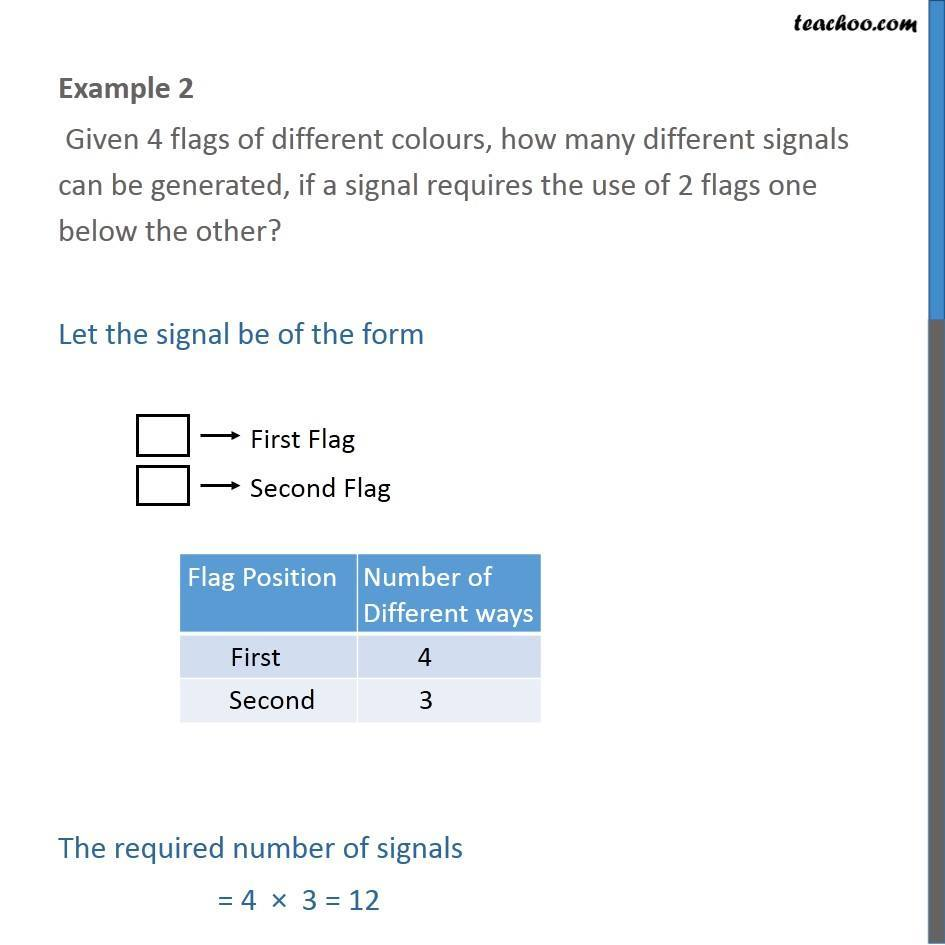1. Chapter 7 Class 11 Permutations and Combinations
2. Serial order wise
3. Examples

Transcript

Example 2 Given 4 flags of different colours, how many different signals can be generated, if a signal requires the use of 2 flags one below the other? Let the signal be of the form The required number of signals = 4 × 3 = 12

Examples